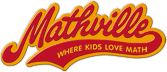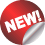#Mathville.com - where kids love math!

These are our newest math games, beginning with the most recently added.

For a complete list, pick "All Math Games by Grade" above.

##Fun Math Learning Games

 Count from 1 to 20 drawing lines from number to number to reveal a mystery picture. Count forward to 20 beginning from a given number within the known sequence (instead of having to begin at 1) to create a mystery picture. Count up to 50 starting at any number less than 50 to make a mystery picture. Count up to 120 starting at any number less than 120 to make a mystery picture. Skip count by 2s or 5s to make a mystery picture. Skip count by 10s to make a mystery picture. Add and subtract within 20 to make a mystery picture. Add and subtract within 100 without regrouping to make a mystery picture. Add and subtract with regrouping to make a mystery picture. Identify triangles, rectangles and squares as parts of a composite shape; recognize partitions of rectangles into equal shares, using the words half, third, and quarter. Work with equal groups of objects to gain foundations for multiplication: Use addition to find the total number of objects arranged in rectangular arrays with up to 5 rows and up to 5 columns. Add within 20 and determine the unknown whole number in an addition equation in a pyramid solitaire card game. Add multiples of 10 within 100, based on an understanding of place value (20 is 2 tens, 30 is 3 tens,... and 100 is 10 tens) - in a pyramid solitaire card game. Given a two-digit number, mentally find 10 more or 10 less than the number to win this classic kids' game. Mentally add or subtract 10 or 100 to or from a given number from 100 to 900 to get three in a row. Know products of two one-digit numbers to develop and carry out a winning strategy. Understand a fraction 1/b as the quantity formed by 1 part when a whole is partitioned into b equal parts. Recognize equivalent common fractions and plan ahead to win this fun solitaire game. Add common fractions to make 1 in a solitaire Dominoes game. Express a fraction with denominator 10 as an equivalent with denominator 100 to add fractions (tenths and hundredths). Add common fractions and mixed numbers and weigh the probabilities. Pair decimal fractions with denominators of 10 or 100 that add to 1. Make valid addition and subtraction statements with decimal tenths and hundredths. Apply knowledge of factors, multiples and divisibility to devise a plan to clear the board. Perform addition and subtraction in the conventional order to arrange numbers to make 6 true equations. Perform multiplication and division in the conventional order to arrange numbers to make 6 true equations.

See All Math Games by Grade

## ClassicVisit Mathville where everything from work to play is filled with math.

©Courseware Solutions Terms of Use and Privacy Policy